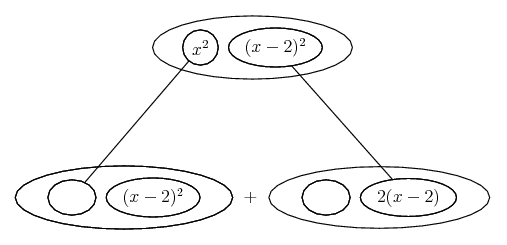# Thread: a curve is defined by y=x^2(x-2)^2

1. ## a curve is defined by y=x^2(x-2)^2

this is the questions: a curve is defined by y=x^2(x-2)^2.show that dy/dx=4x(x-1)(x-2)..

someone please help..

2. Probably easiest to do: product rule then factorise

Edit:

Just in case a picture helps...... where... the product rule. Straight continuous lines differentiate downwards (integrate up) with respect to x

Note that you can almost forget the chain rule for (x-2)^2 because the inner derivative is 1.

__________________________________________

Don't integrate - balloontegrate!

http://www.ballooncalculus.org/forum/top.php

Draw balloons with LaTeX: http://www.ballooncalculus.org/asy/doc.html

#### Search Tags

curve, defined, yx2x22